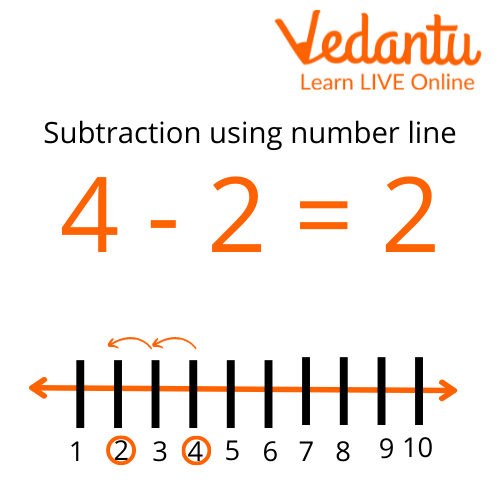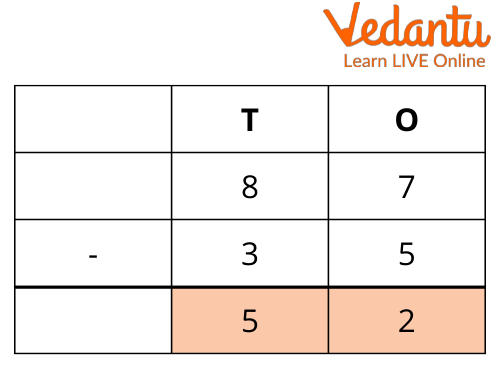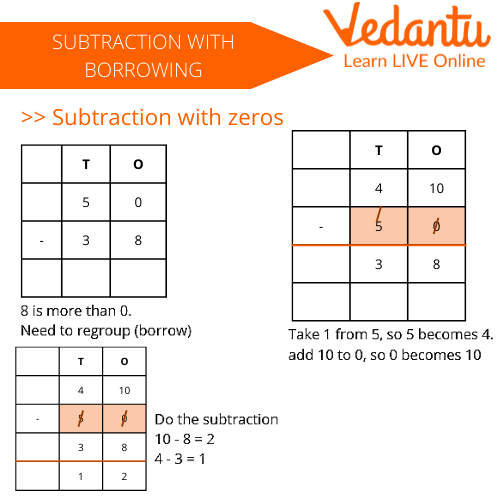Courses
Courses for Kids
Free study material
Free LIVE classes
More

# Subtraction for Class 3LIVE
Join Vedantu’s FREE Mastercalss

## Introduction to Subtraction

If you bought fifty candies in the morning and ate 23 candies at night, then what is the total number of candies left with you? Do you know the answer to this question? Let us learn the concept of subtraction for class 3 with questions and various examples.

The basic concept of subtraction was taught in the previous classes. In subtraction for class 3, we will learn a little advanced concept of subtraction. We will see how to do subtraction sums for class 3 with borrowing. We will be looking at the concept with various examples of subtraction sums. Additional questions will also be provided at the end. Subtraction sums for class 3 will also be shared at the end. So, let us begin the module now.

## Basic Rules of Subtraction

Subtraction has various parts in which it is divided. Minuend, Subtrahend, Minus sign and equals sign are the parts of Subtraction. In the example of 9-8=1, 9 is minuend, 8 is subtrahend, - is the minus sign, and = is the equals sign.

Subtraction is used to calculate the remaining number from the two given numbers. There are certain basic rules to the concept of subtraction. We have to write the two given numbers in a tabular form. The larger number will always be at the top of the smaller number. We always start by subtracting the digits which are on the rightmost side. Further, the tens place digits are subtracted, then the hundredth, and so on.Subtraction through Number Line

## Number Line Representation of Subtraction

Through this number line representation, we can see the basic concept of subtraction. We first mark the larger digit out of the two given numbers. Then we go back to the left side of the line and mark the second digit. After that we count the numbers in between, which is the final answer of the subtraction. 2 is the answer of 4-2.

## Subtraction Without Borrowing

This is a simple form of subtraction. This can be done by simply following the basic rules of subtraction. We keep the larger number at the top and start subtracting by the one's side. Let us take an example to understand this concept.An Example of Subtraction

To subtract 35 from 87, we first subtract 5 from 7 and write the answer 2 in the One's column. After that, we find the subtraction of 3 from 8 and write the answer 5 in the Tens box. This is how a subtraction without borrowing is done.

## Subtraction with Borrowing

In some cases, the numbers to which we have to subtract are smaller. See the example given below, in this case, 8 cannot be subtracted from 0. Hence, let us understand this concept step by step.

• First, start from the One's side and write the digit above it.

• The next step is to take a carry from the tens number which is 5 in this case.

• After taking the carry the number above the one digit will become 10.

• Subtract 8 out of 10 and write the answer 2 below in the column.

• Now that 5 has given 1 carry to 0, only 4 is left.

• Finally, subtract 3 out of 4 and write the answer 1 below.Subtraction Example with Borrowing

## Solved Examples of Subtraction Sums

Q1. At a birthday party, Sonu invited 100 people and only 50 showed up. How many people did not come for the part?Birthday Party

Ans: We solve this kind of question like:

Total number of people Sonu invited = 100

The number of people who showed up = 50

The number of people who did not come= was 100 - 50

The answer would be 50 people.

Q2. A black sheep walks 50 metres in the morning and 40 more in the evening? How many steps did it walk in the evening?Black Sheep

Ans: We solve this kind of question like:

Total number of steps frog walks in the morning = 50

Number of frog walks in the evening = 40

Number of steps it walked less in the evening = 50 - 40

The answer would be 10 steps.

(Subtraction sums for class 3 with borrowing)

Q1: 672 - 563=?

Ans: 109

Q2: 432-234=?

Ans: 198

## Summary

Subtraction is a very important concept for the students to make their base level strong. These concepts will always be helpful in all kinds of theoretical and practical questions. The advanced forms of subtraction are added class by class. Students must apply these rules in their daily lives as well. Whenever they go to buy snacks or candies, they must use these concepts. They should also practice more and more worksheets for a better understanding. These are a good form of understanding.

Last updated date: 15th Sep 2023
Total views: 113.1k
Views today: 1.13k

## FAQs on Subtraction for Class 3

1.  What are parts of subtraction?

Subtraction is used to subtract a smaller number from a larger number. It is used in various concepts of mathematics. Minuend, Subtrahend, Minus sign and equals sign are the parts of a subtraction. Let us take an example to understand the parts.

In questions 7-3=4, 7 is minuend, 3 is subtrahend, - is a minus sign, and = is an equals sign. These parts form a subtraction.

2.  How to solve word problem-based subtraction questions? Give an example for the same.

Word problem-based subtraction questions are the advanced form of subtraction. Let's take an example to understand this better. If a rabbit has 22 carrots and eats 12  out of them. How many carrots are left with the rabbit, to answer this question let us go step by step.

The total number of carrots a rabbit has = 22

Number of carrots rabbit has already eaten= 12

The number of carrots left with the rabbit is = 22 - 12= 10.